RRB JE ME (CBT I) Mock Test- 9

# RRB JE ME (CBT I) Mock Test- 9

Test Description

## 100 Questions MCQ Test RRB JE Mock Test Series for Mechanical Engineering (ME) | RRB JE ME (CBT I) Mock Test- 9

RRB JE ME (CBT I) Mock Test- 9 for Railways 2023 is part of RRB JE Mock Test Series for Mechanical Engineering (ME) preparation. The RRB JE ME (CBT I) Mock Test- 9 questions and answers have been prepared according to the Railways exam syllabus.The RRB JE ME (CBT I) Mock Test- 9 MCQs are made for Railways 2023 Exam. Find important definitions, questions, notes, meanings, examples, exercises, MCQs and online tests for RRB JE ME (CBT I) Mock Test- 9 below.
Solutions of RRB JE ME (CBT I) Mock Test- 9 questions in English are available as part of our RRB JE Mock Test Series for Mechanical Engineering (ME) for Railways & RRB JE ME (CBT I) Mock Test- 9 solutions in Hindi for RRB JE Mock Test Series for Mechanical Engineering (ME) course. Download more important topics, notes, lectures and mock test series for Railways Exam by signing up for free. Attempt RRB JE ME (CBT I) Mock Test- 9 | 100 questions in 90 minutes | Mock test for Railways preparation | Free important questions MCQ to study RRB JE Mock Test Series for Mechanical Engineering (ME) for Railways Exam | Download free PDF with solutions
 1 Crore+ students have signed up on EduRev. Have you?
RRB JE ME (CBT I) Mock Test- 9 - Question 1

### If the rate of interest is 8% per annum and Rs. 10000 lent at the compound interest half yearly then calculate the equivalent simple rate of interest for the first year?

Detailed Solution for RRB JE ME (CBT I) Mock Test- 9 - Question 1 Let the principal be Rs.100

the rate of interest is 8% per annum lent at the compound interest half yearly.

Compound interest for 6 months = 4%

Compound interest next 6 months = 4% + 4of 4% = (4 +.16)% = 4.16%

the equivalent simple rate of interest for the first year = 4 + 4.16 = 8.16%

RRB JE ME (CBT I) Mock Test- 9 - Question 2

### Three taps A, B, and C can fill a tank in 20, 24, and 30 hours, respectively. How long (in hours) would the three taps take to fill the tank if all of them are opened together?

Detailed Solution for RRB JE ME (CBT I) Mock Test- 9 - Question 2 A can fill 1 unit of water in the tank in 1/20 hours

B can fill 1 unit of water in tank in 1/24 hours

C can fill 1 unit of water in tank in 1/30 hours

(A+B+C) can fill 1 unit of water in tank in 1/20+1/24+1/30 = 6+5+4/120 = 15/120 = 18 days

Therefore,

(A+B+C) can fill full tank in 8 days.

RRB JE ME (CBT I) Mock Test- 9 - Question 3

### PAB and PCD are two secants to a circle. if PA =10cm , AB =12cm and PC =11cm, then what is the value of PD?

Detailed Solution for RRB JE ME (CBT I) Mock Test- 9 - Question 3

PA x PB = PC x PD

Let PD = X cm

10 x (10 + 12) = 11 x (11 + X)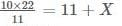11 + X = 20

X = 9

PD = 9 = 11 = 20 cm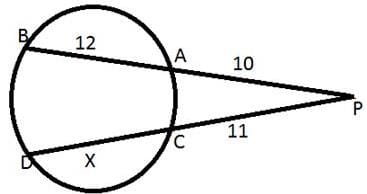RRB JE ME (CBT I) Mock Test- 9 - Question 4

The speed of a boat in still water is 10 km/hr. It covers a distance of 50 km upstream in 6 hours. What is the speed (in km/hr) of the stream?

Detailed Solution for RRB JE ME (CBT I) Mock Test- 9 - Question 4 Speed of boat = 10 km/hr

Upstream speed 50/6 = 25/3 km/hr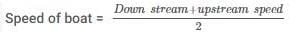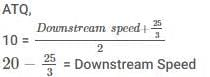So, Downstream speed 35/3 km/hr.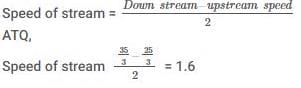RRB JE ME (CBT I) Mock Test- 9 - Question 5

500 litres of milk solution has 40% milk in it. How much more milk should be added to make it 60% in the solution?

Detailed Solution for RRB JE ME (CBT I) Mock Test- 9 - Question 5 Milk = 40% of 500 = 200 litres

Water is 60% of 500 = 300 litres

ATQ,

40%of mixture = water = 300 litres

New mixture quantity = 300/40% = 750 liters

The quantity of milk in the mixture = 60 % of 750 = 450 liters

Required milk to be mixed = 450 - 200 = 250 liters

Therefore,

250 liter of milk should be added to make it 60% in the solution.

RRB JE ME (CBT I) Mock Test- 9 - Question 6

The shadow of a tower on the ground level is increased by 20 m, when the angle of depression by the sun changes from 60 to 30. The height of the tower is?

Detailed Solution for RRB JE ME (CBT I) Mock Test- 9 - Question 6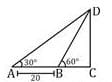Let the height of tower DC = h meter

tan 600 = h/BC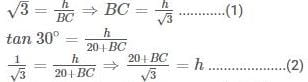Putting the value of BC from equation(l) in equation (2), we get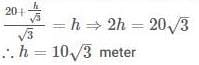RRB JE ME (CBT I) Mock Test- 9 - Question 7

What will be the sum of all odd whole numbers between 10 and 38?

Detailed Solution for RRB JE ME (CBT I) Mock Test- 9 - Question 7 To calculate sum, we need to find the number of odd whole numbers between 10 and 38 using the Arithmetic Progression formula.

That is,

Last term = First term + (number of terms-1) × Common difference

ATQ,

37 = 11 + (N-1) × 2

So, N or Number of terms = 14

Now,

Sum of terms = N/2 [2×first term(N−1)×d]

ATQ,

Sum = 14/2 [2×11+(14−1)×2]

Sum = 336

Therefore,

The Sum of all odd whole numbers between 10 and 38 is 336

RRB JE ME (CBT I) Mock Test- 9 - Question 8

If the volume of two cubes are in the ratio 125 : 1, then what is the ratio of their edges?

Detailed Solution for RRB JE ME (CBT I) Mock Test- 9 - Question 8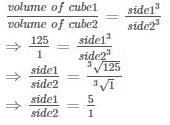RRB JE ME (CBT I) Mock Test- 9 - Question 9

A , B, and C invest in a business in the ratio 3:6:5. A and C are working partners. Only B is a sleeping partner; hence his share will be 3/4th of what it would have been if he were a working partner. If they make Rs. 50000 profit, half of which is reinvested in the business and the other half is distributed between partners, then how much does C get (in Rs)?

Detailed Solution for RRB JE ME (CBT I) Mock Test- 9 - Question 9

Given the investment ratio old ratio

A : B: C

3 : 6 : 5

ATQ,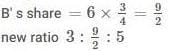total profit = 50000

after reinvestment the profit

= 50000/2 = 25000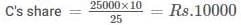RRB JE ME (CBT I) Mock Test- 9 - Question 10

The HCF and LCM of the two numbers are 45 and 270, respectively. If the ratio of the two numbers is 2:3 and the smaller of the two numbers is X, then find X2.

Detailed Solution for RRB JE ME (CBT I) Mock Test- 9 - Question 10 We know that

Product of numbers = product of lcm and hcf

2y × 3y = 45 × 270

y2 = 45 × 45

y = 45

then smaller no.

X = 2 × 45 = 90

X2 = (90)2 = 8100

RRB JE ME (CBT I) Mock Test- 9 - Question 11

What is the value of cos40− cos140/sin80+ sin120?

Detailed Solution for RRB JE ME (CBT I) Mock Test- 9 - Question 11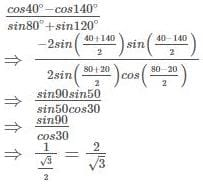RRB JE ME (CBT I) Mock Test- 9 - Question 12

What is the value of tan (π/4 + A) × tan (3π/4 + A)?

Detailed Solution for RRB JE ME (CBT I) Mock Test- 9 - Question 12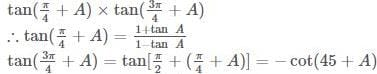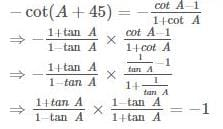RRB JE ME (CBT I) Mock Test- 9 - Question 13

The distance between the centers of the two circles is 61cm and their radius is 35cm and 24cm. What is the length(in cm) of the direct common tangent to the circles?

Detailed Solution for RRB JE ME (CBT I) Mock Test- 9 - Question 13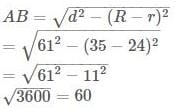RRB JE ME (CBT I) Mock Test- 9 - Question 14

What is the value of 2cot P−A/2/1+tan2 2P−A/2 ?

Detailed Solution for RRB JE ME (CBT I) Mock Test- 9 - Question 14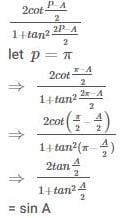RRB JE ME (CBT I) Mock Test- 9 - Question 15

Find the standard deviation of {11, 7, 10, 13, 9}

Detailed Solution for RRB JE ME (CBT I) Mock Test- 9 - Question 15 First we have to find the mean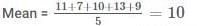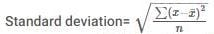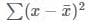= (11 - 10)2 + (7 - 10)2 + (10 - 10)2 + (13 - 10)2 + (9 - 10)2

= 1 + 9 + 0 + 9 + 1 = 20

Standard deviation =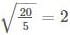RRB JE ME (CBT I) Mock Test- 9 - Question 16

If a dairy mixes cow’s milk which contains 10%fat with buffalo’s milk which contains 20% fat,then the resulting mixture has the fat of 120/7%. In what ratio was the cow’s milk mixed with buffalo’s milk?

Detailed Solution for RRB JE ME (CBT I) Mock Test- 9 - Question 16

Use alligation rule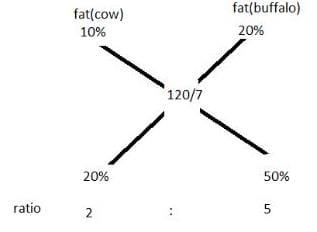RRB JE ME (CBT I) Mock Test- 9 - Question 17

If two complementary angles are in the ratio of 4:5, find the greater angle.

Detailed Solution for RRB JE ME (CBT I) Mock Test- 9 - Question 17 Let the angles be 4x , 5x

4x + 5x = 90

9x = 90

x = 10

Greater angle = 5x = 50

RRB JE ME (CBT I) Mock Test- 9 - Question 18

If cosθ = 4/5, then (secθ+cosecθ)=

Detailed Solution for RRB JE ME (CBT I) Mock Test- 9 - Question 18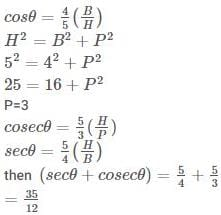RRB JE ME (CBT I) Mock Test- 9 - Question 19

In the given figure, in a right angle triangle ABC, AB = 12 cm and AC = 15cm. A square is inscribed in the triangle. One of the vertices of the square coincides with the vertex of the triangle. What is the maximum possible area of the square (cm2)?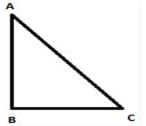Detailed Solution for RRB JE ME (CBT I) Mock Test- 9 - Question 19

AB = 12 cm, AC = 15 cm

then, BC = 152 - 122 = 9cm

side of square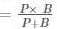where, P = perpendicular, B = base

side of the square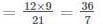area of the largest square that can be formed

inside the triangle = (36/7)2 = 1296/49

RRB JE ME (CBT I) Mock Test- 9 - Question 20

What is the measure of the two equal angles of a right isosceles triangle?

Detailed Solution for RRB JE ME (CBT I) Mock Test- 9 - Question 20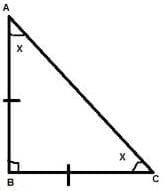A.T.Q

∠B = 90°

and AB = BC

So, ∠A = ∠C

we know that

∠A + ∠B + ∠C = 180°

x + 90° + x = 180°

2x = 90°

x = 45°

RRB JE ME (CBT I) Mock Test- 9 - Question 21

If 1082 = 11664, then what is the value of √1.1664 + √116.64 ?

Detailed Solution for RRB JE ME (CBT I) Mock Test- 9 - Question 21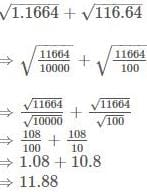RRB JE ME (CBT I) Mock Test- 9 - Question 22

The length and breadth of a rectangular field are in the ratio of 5 : 2. If the perimeter of the field is 140 metres, then what is the breadth (in metres)?

Detailed Solution for RRB JE ME (CBT I) Mock Test- 9 - Question 22 Let Length and Breadth of rectangle are 5x and 2x respectively.

140 = 2(5x+2x)

70 = 7x

So, x = 10 m

Therefore, Breadth of rectangle = 2×10=20 metre

RRB JE ME (CBT I) Mock Test- 9 - Question 23

Calculate the amount on Rs. 37,500 @ 8% p.a compounded half yearly for 1 1/2 years.

Detailed Solution for RRB JE ME (CBT I) Mock Test- 9 - Question 23

As the interest compounded half yearly

So time will be =3 half year

Rate = 8/2 = 4%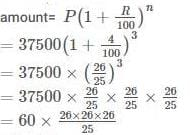= 42182.4

RRB JE ME (CBT I) Mock Test- 9 - Question 24

The mean of 17 numbers is 12. If two numbers 9 and 15 are removed, then what will be the mean of remaining number?

Detailed Solution for RRB JE ME (CBT I) Mock Test- 9 - Question 24 Sum of 17 numbers = 17 ×12 = 204

After removal of 9 and 15, sum becomes 180.

Therefore,

Mean of remaining 15 numbers = 180/15=12

RRB JE ME (CBT I) Mock Test- 9 - Question 25

Ram Naresh got two successive discounts, a bag with a list price of Rs. 400 is available at Rs. 160. If the second discount is 20%, find the first discount.

Detailed Solution for RRB JE ME (CBT I) Mock Test- 9 - Question 25

Let the first discount be x%

First a discount of 20% means that you multiply the price by. 80(1 - 0.20)

The initial price is therefore first multiplied by x, then by 0.80, and the result is 160.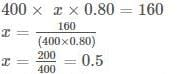Multiply by 0.5 means reduce by 50%.

RRB JE ME (CBT I) Mock Test- 9 - Question 26

If the diagonal of a square is 12 cm, then what is the area (in cm2) of the square?

Detailed Solution for RRB JE ME (CBT I) Mock Test- 9 - Question 26

Diagonal of square = side x √2

Area of square = side2

ATQ,

Diagonal = 12cm

Side x √2 = 12

So, side = 12/√2

Therefore,

Area = (12/√2)2 ⇒ 144/2 ⇒ 72

RRB JE ME (CBT I) Mock Test- 9 - Question 27

A line passing through (3, 5) makes the angle of 135 with x-axis. What will be its equation?

Detailed Solution for RRB JE ME (CBT I) Mock Test- 9 - Question 27

Slope = tan 1350 = −1 = m

⇒ A (3,5)

(y - 5) = -(x - 3)

Equation of line

x + y - 8 = 0

RRB JE ME (CBT I) Mock Test- 9 - Question 28

Three toys are in the shape of the cylinder, hemisphere, and cone . The three toys have the same base. The height of each toy is 2 √2. What is the ratio of the total surface areas of the cylinder, hemisphere and cone respectively ?

Detailed Solution for RRB JE ME (CBT I) Mock Test- 9 - Question 28

Height of each toy = 2√2

since height of hemisphere = radius of hemisphere

= radius of each toy = 2√2

slant height of cone(l)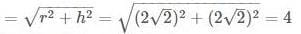ratio = T.S.A of cylinder: T.S.A of the hemisphere : T.S.A of cone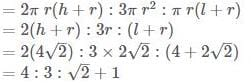RRB JE ME (CBT I) Mock Test- 9 - Question 29

A sum of Rs 4000 becomes Rs 5800 in 3 years, when invested in a scheme of simple interest. If the same sum is invested in a scheme of compound interest with same yearly interest rate (compounding of interest is done yearly), then what will be the amount (in Rs) after 2 years?

Detailed Solution for RRB JE ME (CBT I) Mock Test- 9 - Question 29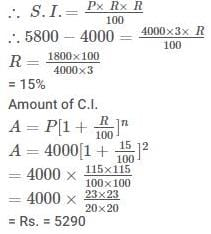RRB JE ME (CBT I) Mock Test- 9 - Question 30

Average of the runs of 133 players of a team is 38. If the average of the runs of the male players is 43 and the average of the runs of the female players is 24, then what will be the ratio of the total runs of male players and the total runs of female players respectively?

Detailed Solution for RRB JE ME (CBT I) Mock Test- 9 - Question 30 The ratio of male females can be calculated by the formula of the allegation.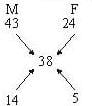M :F = 1 4 :1 0 = 14 : 5

No. of males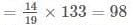Total runs of males

= 98 x 43

No. of females

= 133 - 9 8 = 35

Total runs of females

= 35 x 24

Required ratio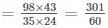RRB JE ME (CBT I) Mock Test- 9 - Question 31

D said A’s father is the only brother of my sister’s son. How is A’s father related to D?

Detailed Solution for RRB JE ME (CBT I) Mock Test- 9 - Question 31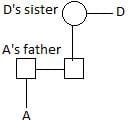A’s father is the nephew of D. Hence option (b) is correct.

RRB JE ME (CBT I) Mock Test- 9 - Question 32

In the given sequence how many such symbols and numbers are there which are either immediately preceded or immediately followed by the letters which is from the first half of the English alphabet?

2 L K @ 8 \$ P B 1 V # 6 % G W 9 J C D 4 © 7 F R 4 A

Detailed Solution for RRB JE ME (CBT I) Mock Test- 9 - Question 32 Such combinations are

2L, K@, B1, %G, 9J, D4, 7F, 4A,

RRB JE ME (CBT I) Mock Test- 9 - Question 33

In the following questions, find out the answer figure which completes the problem figure matrix.

Problem Figure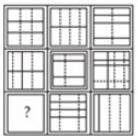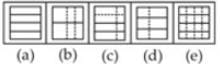Detailed Solution for RRB JE ME (CBT I) Mock Test- 9 - Question 33 Each row and column contains six complete lines and six broken lines.
RRB JE ME (CBT I) Mock Test- 9 - Question 34

Arrange the given words in the sequence in which they occur in the dictionary.

1. Crown

2. Custom

3. Cable

4. Cupid

5. Creep

Detailed Solution for RRB JE ME (CBT I) Mock Test- 9 - Question 34 The words are arranged in the dictionary order as follows:-

3. Cable

5. Creep

1. Crown

4. Cupid

2. Custom

RRB JE ME (CBT I) Mock Test- 9 - Question 35

In the questions given below, some relationship has been expressed through symbols as shown below. Based on the meaning of these symbols and choose the correct answer.

ϕ means ‘less than.’

Δ means ‘not greater than.’

- means ‘equal to.’

+ means ‘not equal to.’

× means ‘not less than.’

= means ‘greater than.’

X - Y = Z implies

Detailed Solution for RRB JE ME (CBT I) Mock Test- 9 - Question 35 Clearly, the meaning of the given symbols

X - Y = Z ⇒ X = Y > Z

Using the proper notations/symbols in option (b), we get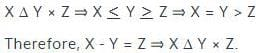RRB JE ME (CBT I) Mock Test- 9 - Question 36

Find the missing term.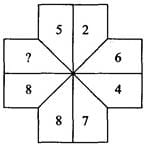Detailed Solution for RRB JE ME (CBT I) Mock Test- 9 - Question 36

There are two alternate series.

1st series: 5, 6, 7, 8, 9, ... and so on.

2nd series: 2 (×2), 4 (×2), 8 (×2) ... and so on.

RRB JE ME (CBT I) Mock Test- 9 - Question 37

How many hexagons are their in the figure given below?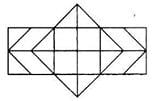Detailed Solution for RRB JE ME (CBT I) Mock Test- 9 - Question 37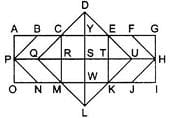∴ Total hexagons = CDEKLM, CEUKMQ, CFHJMQ, BEUKNP, BFHJNP = 5

RRB JE ME (CBT I) Mock Test- 9 - Question 38

‘Suma’ is shorter than ‘Uma’, ‘Neha is taller then ‘Suma’, ‘Sudha’ is taller than ‘Uma’ but shorter than ‘Hema’. ‘Uma’ is taller than ‘Neha’. Who is the tallest among them?

Detailed Solution for RRB JE ME (CBT I) Mock Test- 9 - Question 38 Uma > Suma ---------------- (i)

Neha > Suma ------------------(ii)

Hema > Sudha > Uma > Neha ---------(iii)

On combining above statement,

Hema > Sudha > Uma > Neha > Suma

Therefore, Hema is the tallest among all.

RRB JE ME (CBT I) Mock Test- 9 - Question 39

A word is represented by only one set of numbers as given in anyone of the alternatives. Two alphabets classes represent the set of numbers given in the alternatives, as in the 2 matrices given below. The columns and rows of Matrix I are from 0 to 4 and Matrix II from 5 to 9. A letter can be represented first by its row and next by its column number. e.g., ‘C’ can be represented by 02, 21, etc. ‘T’ can be represented by 65, 96, etc. Similarly, you have to identify the set of words for ‘STAMP.’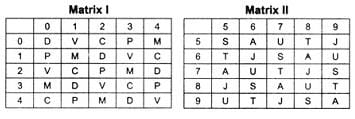Detailed Solution for RRB JE ME (CBT I) Mock Test- 9 - Question 39 According to the matrices,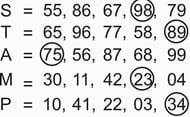∴ Stamp = 98, 89, 75, 23, 34

RRB JE ME (CBT I) Mock Test- 9 - Question 40

Choose the odd one out of the given alternatives.

Detailed Solution for RRB JE ME (CBT I) Mock Test- 9 - Question 40 In all other groups there is a gap of one letter as in the alphabet between first and third letter.
RRB JE ME (CBT I) Mock Test- 9 - Question 41

DRIVEN is related to EIDRVN in the same way as BEGUM is related to ________.

Detailed Solution for RRB JE ME (CBT I) Mock Test- 9 - Question 41

As,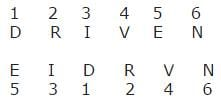Similarly,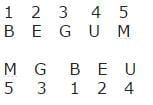RRB JE ME (CBT I) Mock Test- 9 - Question 42

Choose the odd one out from the given alternatives.

Detailed Solution for RRB JE ME (CBT I) Mock Test- 9 - Question 42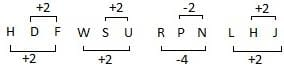RRB JE ME (CBT I) Mock Test- 9 - Question 43

Select the related word from the give alternatives.

BCG : URP :: LMQ : ???

Detailed Solution for RRB JE ME (CBT I) Mock Test- 9 - Question 43 The series will be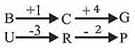Same way,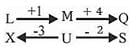RRB JE ME (CBT I) Mock Test- 9 - Question 44

If HOSPITAL is written as 32574618 in a certain code, how would POSTAL be written in that code ?

Detailed Solution for RRB JE ME (CBT I) Mock Test- 9 - Question 44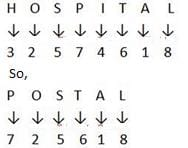RRB JE ME (CBT I) Mock Test- 9 - Question 45

Three of the following four are alike in a certain way and hence form a group. Which is the one that does not belong to that group?

Detailed Solution for RRB JE ME (CBT I) Mock Test- 9 - Question 45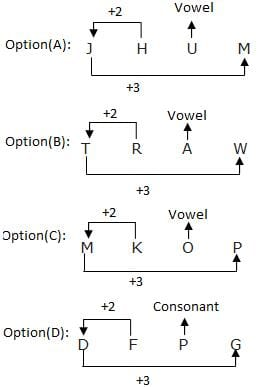Here option (D) does not belong to the group.

RRB JE ME (CBT I) Mock Test- 9 - Question 46

In the question below, there are few statements followed by few conclusions. You have to take the given statements to be true even if they seem to be at variance with commonly known facts and then decide which of the given conclusion logically follow(s) from the given statements.

Statements:

1. Some books are pens.

2. Some pens are pencils.

3. All pencils are rubber.

Conclusions:

I. Some books are pencils.

II. No book is a pencil.

Detailed Solution for RRB JE ME (CBT I) Mock Test- 9 - Question 46 Either conclusion I or II follows.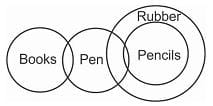RRB JE ME (CBT I) Mock Test- 9 - Question 47

Identify the diagram that best represents the relationship among Iron, Lead, Nitrogen.

Choose the correct venn diagram.

Detailed Solution for RRB JE ME (CBT I) Mock Test- 9 - Question 47 There is no relation between iron, lead and nitrogen.

So,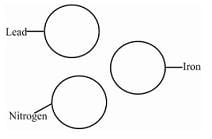RRB JE ME (CBT I) Mock Test- 9 - Question 48

In the following questions, a word is followed by five other words, one of which cannot be formed by using the letters of the given the word. Find this word

CLASSIFICATION

Detailed Solution for RRB JE ME (CBT I) Mock Test- 9 - Question 48 As there is only one ‘N’ letter in the given word
RRB JE ME (CBT I) Mock Test- 9 - Question 49

If in a certain code language ‘CONTROL’ is written as ‘DMQPWIS’, then how will ‘BROTHQR’ be written in that code?

Detailed Solution for RRB JE ME (CBT I) Mock Test- 9 - Question 49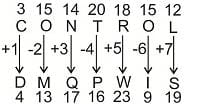Similarly,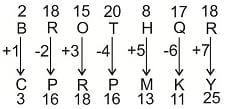RRB JE ME (CBT I) Mock Test- 9 - Question 50

Mohan starts walking East and walked 30 metres, then he turned right and walked 50 metres and he again turned left and walked 40 metres. Again he turned left and walked 50 metres. Now, how far is he from his starting point?

Detailed Solution for RRB JE ME (CBT I) Mock Test- 9 - Question 50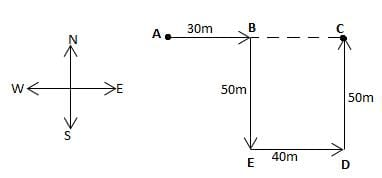Start point is A

End point is C

AC = AB + BC

= 30 + 40

= 70 (BC = ED)

RRB JE ME (CBT I) Mock Test- 9 - Question 51

Solve the following series?

B2F, G4K, L12P, Q38U, ?

Detailed Solution for RRB JE ME (CBT I) Mock Test- 9 - Question 51 The series is as follows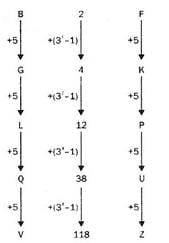V118Z will come in place of ‘?’.

RRB JE ME (CBT I) Mock Test- 9 - Question 52

Find the odd one out from the given alternatives.

Detailed Solution for RRB JE ME (CBT I) Mock Test- 9 - Question 52 In all other groups there is a gap of two letters as in alphabet between third and fourth letter.
RRB JE ME (CBT I) Mock Test- 9 - Question 53

What should come at the place of question mark?

2, A, 9, B, 6, C, 13, D, ?

Detailed Solution for RRB JE ME (CBT I) Mock Test- 9 - Question 53

Here,

Series I = 2, 9, 6, 13, ?

Series II = A, B, C, D

In Series I,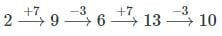RRB JE ME (CBT I) Mock Test- 9 - Question 54

A and B are sisters. R and S are brothers. A’s daughter is R’s sister. What is B’s relation to S?

Detailed Solution for RRB JE ME (CBT I) Mock Test- 9 - Question 54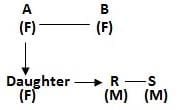Clearly B is the aunt of S.

RRB JE ME (CBT I) Mock Test- 9 - Question 55

There are two sets of figures namely problem Figure containing five figures 1, 2, 3, 4, 5 and answer figures (a), (b), (c), (d). You have to select one Figure from the answer set which will continue the same series as given in problem set figures.

Problem figure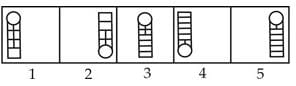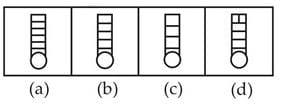Detailed Solution for RRB JE ME (CBT I) Mock Test- 9 - Question 55 Problem figure 2 is reverse of figure 1 with same number of horizontal lines and vertical lines decreases by one row. Similarly, Figure 4 is revers of figure 3 and symmetrical changes in pattern of lines. Therefore, answer figure (a) is reverse of problem figure 5.
RRB JE ME (CBT I) Mock Test- 9 - Question 56

Through which of the following states does the river Chambal flow?

Detailed Solution for RRB JE ME (CBT I) Mock Test- 9 - Question 56 The river Chambal flows through U.P., M.P., Rajasthan
RRB JE ME (CBT I) Mock Test- 9 - Question 57

Radcliffe Line was the boundary demarcation line between India and ___________

Detailed Solution for RRB JE ME (CBT I) Mock Test- 9 - Question 57 Radcliffe Line was the boundary demarcation line between India and Pakistan.
RRB JE ME (CBT I) Mock Test- 9 - Question 58

How many members of the Rajya Sabha retire from the house every 2 years?

Detailed Solution for RRB JE ME (CBT I) Mock Test- 9 - Question 58 The Rajya Sabha is also known as the “Council of States” or the upper house. Rajya Sabha is a permanent body and is not subject to dissolution. However, one-third of the members retire every second year and are replaced by newly elected members.
RRB JE ME (CBT I) Mock Test- 9 - Question 59

Which country recently launched its first satellite for space-based broadband to rival Google?

Detailed Solution for RRB JE ME (CBT I) Mock Test- 9 - Question 59 China, on December 22, 2018, launched its first communication satellite to provide broadband internet services worldwide in an apparent bid to rival Google and other international firms. The satellite is the first Hongyun project planned by China Aerospace Science and Industry Corp (CASIC).
RRB JE ME (CBT I) Mock Test- 9 - Question 60

Which of the following sultans used to patronize Minhaj-us-Siraj?

Detailed Solution for RRB JE ME (CBT I) Mock Test- 9 - Question 60 Iltutmish had patronized Minhaj-us-Siraj
RRB JE ME (CBT I) Mock Test- 9 - Question 61

Whom does the President of India send his resignation if he wants to quit his office?

Detailed Solution for RRB JE ME (CBT I) Mock Test- 9 - Question 61 President of India send his resignation to the Vice-President of India if he wants to quit his office
RRB JE ME (CBT I) Mock Test- 9 - Question 62

Sultana Razia Begum was the daughter of:-

Detailed Solution for RRB JE ME (CBT I) Mock Test- 9 - Question 62 She was the sultan of Delhi from 1236 - 1240. Razia Sultan was the only women ruler of both the sultanate & Mughal periods.
RRB JE ME (CBT I) Mock Test- 9 - Question 63

The book “The God of small things” is written by :

Detailed Solution for RRB JE ME (CBT I) Mock Test- 9 - Question 63 Arundhati Roy (born 24 November 1961) is an Indian author and political activist who was best known for the 1998 Man Booker Prize for ‘Fiction winning novel The God of Small Things (1997) and for her involvement in environmental and human rights causes. Roy’s novel became the biggest-selling book by a non-expatriate Indian author. Roy began writing her first novel, The God of small Thins, in 1992, completing it in 1996. The book is semi-autobiographical and a major part captures her childhood experiences in Aymanam. The publication of The God of Small Things catapulted Roy to instant international fame. It received the 1997 Booker Prize for Fiction and was listed as one of the New York Times Notable Books of the Year for 1997. It reached fourth position on the New York Times Bestsellers list for Independent Fiction.
RRB JE ME (CBT I) Mock Test- 9 - Question 64

What is the rank of TCS in 100 most valuable U.S. brands

Detailed Solution for RRB JE ME (CBT I) Mock Test- 9 - Question 64 Tata Consultancy Services Ltd (TCS) has been ranked among the top 100 most valuable U.S. brands (58th) for the fourth consecutive year by Brand Finance, a leading brand valuation firm.
RRB JE ME (CBT I) Mock Test- 9 - Question 65

Who was the Chairman of the first Finance Commission?

Detailed Solution for RRB JE ME (CBT I) Mock Test- 9 - Question 65 K C Niyogi was the Chairman of the first Finance Commission from 1952-57
RRB JE ME (CBT I) Mock Test- 9 - Question 66

Which of the following gases has the highest concentration in the atmosphere after nitrogen?

Detailed Solution for RRB JE ME (CBT I) Mock Test- 9 - Question 66 After nitrogen, oxygen has the highest concentration of 20.95 percent in the Earth’s atmosphere
RRB JE ME (CBT I) Mock Test- 9 - Question 67

Which of the following countries has become the second country to legalize the use of cannabis?

Detailed Solution for RRB JE ME (CBT I) Mock Test- 9 - Question 67 Canada has become the second country after Uruguay to legalise possession and use of recreational cannabis.
RRB JE ME (CBT I) Mock Test- 9 - Question 68

The Qutub Minar was completed by the famous ruler.

Detailed Solution for RRB JE ME (CBT I) Mock Test- 9 - Question 68 The Construction of Qutub Minar was started by Qutub-ud-din Aibak. Construction of Qutub Minar was started by Qutub ud-din Aibek, the first ruler of the Delhi Sultanate. In the memory of Qutbuddin Bakhtiar Kaki, the famous Sufi saint. But after his sudden death, his son-in-law Illtutmish completed it. In 1368 A.D., the top storey was damaged by lightning and was rebuilt by Firoz Shah Tughlaq.
RRB JE ME (CBT I) Mock Test- 9 - Question 69

Who has signed an MoU for establishing ROSHNI-Centre of Women Collectives led Social Action with Deendayal Antyodaya Yojana – National Rural Livelihood Mission (DAY-NRLM) and Ministry of Rural Development?

Detailed Solution for RRB JE ME (CBT I) Mock Test- 9 - Question 69 Deendayal Antyodaya Yojana – National Rural Livelihood Mission (DAY-NRLM), Ministry of Rural Development and Lady Irwin College, have signed a Memorandum of Understanding (MoU) for establishing ROSHNI – Centre of Women Collectives led Social Action.
RRB JE ME (CBT I) Mock Test- 9 - Question 70

Jama Masjid was was built by -

Detailed Solution for RRB JE ME (CBT I) Mock Test- 9 - Question 70 Jama Masjid was was built by Shah Jahan.
RRB JE ME (CBT I) Mock Test- 9 - Question 71

Who gave the Universal law of gravitation?

Detailed Solution for RRB JE ME (CBT I) Mock Test- 9 - Question 71 Newton gave the Universal law of gravitation
RRB JE ME (CBT I) Mock Test- 9 - Question 72

Electron volt (eV) is a unit of

Detailed Solution for RRB JE ME (CBT I) Mock Test- 9 - Question 72 Electron volt (eV) is a unit of Energy.
RRB JE ME (CBT I) Mock Test- 9 - Question 73

What is the directional growth movement of a plant part in response to the touch of an object called?

Detailed Solution for RRB JE ME (CBT I) Mock Test- 9 - Question 73 Thigmotropism is the directional response of a plant organ to touch or physical contact with a solid object this directions response is generally caused by the induction of some pattern of differential growth. This phenomenon Is clearly illustrated by the climbing tendrils of some plants, such as the sweet pea. The tendrils actually “feel the solid object, which results in the coiling response
RRB JE ME (CBT I) Mock Test- 9 - Question 74

Which of the following colour will scatter the most while passing through a prism?

Detailed Solution for RRB JE ME (CBT I) Mock Test- 9 - Question 74 Lights of different colour depending upon their scattering in ascending ordor are violet, indigo, blue, green, yellow, orange and red.
RRB JE ME (CBT I) Mock Test- 9 - Question 75

The chemical behaviour of an atom is determined by its

Detailed Solution for RRB JE ME (CBT I) Mock Test- 9 - Question 75 The chemical behaviour of an atom is determined by its Atomic number because that indicates the number of electrons.
RRB JE ME (CBT I) Mock Test- 9 - Question 76

Which vitamin is prepared by our body in the presence of sunlight?

Detailed Solution for RRB JE ME (CBT I) Mock Test- 9 - Question 76 Vitamin D.-Vitamin D can be made by our body itself. Our skin uses sunlight to produce vitamin D.
RRB JE ME (CBT I) Mock Test- 9 - Question 77

Light from the sun reaches Earth upon in nearly

Detailed Solution for RRB JE ME (CBT I) Mock Test- 9 - Question 77 Light from the sun reaches Earth upon in nearly 8 minute.
RRB JE ME (CBT I) Mock Test- 9 - Question 78

All Enzymes are actually ____

Detailed Solution for RRB JE ME (CBT I) Mock Test- 9 - Question 78 Protein

Most enzymes are proteins, although a few are catalytic RNA molecules.

RRB JE ME (CBT I) Mock Test- 9 - Question 79

Ozone layer is present in ____

Detailed Solution for RRB JE ME (CBT I) Mock Test- 9 - Question 79 The ozone layer is present in the stratosphere.
RRB JE ME (CBT I) Mock Test- 9 - Question 80

Soda water contains____

Detailed Solution for RRB JE ME (CBT I) Mock Test- 9 - Question 80 Carbon dioxide

Carbonated water, also known as sparkling water, seltzer water, seltzer or the closely related club soda or soda water into which carbon dioxide gas under pressure has been dissolved.

RRB JE ME (CBT I) Mock Test- 9 - Question 81

Cockroach is a:

Detailed Solution for RRB JE ME (CBT I) Mock Test- 9 - Question 81 Cockroaches are omnivores and can eat almost any type of organic material to survive, which makes them one of the healthiest insects on the planet. Although they prefer sweets, meats and starches, they are also known to consume other items such as hair, books and decaying matter.
RRB JE ME (CBT I) Mock Test- 9 - Question 82

Within elastic limit, the ratio of lateral strain to the linear strain is known as

Detailed Solution for RRB JE ME (CBT I) Mock Test- 9 - Question 82 Within elastic limit, the ratio of lateral strain to the linear strain Is known as Poisson’s ratio
RRB JE ME (CBT I) Mock Test- 9 - Question 83

The lowest frequency Range is of __________in the radio spectrum ?.

Detailed Solution for RRB JE ME (CBT I) Mock Test- 9 - Question 83 L-Band refers to the operating frequency range of 1–2 GHz in the radio spectrum. The wavelength range of L band is 30–15 cm. The L band is one of the chief operating ranges used by various applications such as radars, global positioning systems (GPS), radio, telecommunications and aircraft surveillance
RRB JE ME (CBT I) Mock Test- 9 - Question 84

1 pound is equal to …………….

Detailed Solution for RRB JE ME (CBT I) Mock Test- 9 - Question 84 1 pound is equal to 0.4536 g.
RRB JE ME (CBT I) Mock Test- 9 - Question 85

Due to which of the following are oversized holes in drilling caused?

Detailed Solution for RRB JE ME (CBT I) Mock Test- 9 - Question 85 oversized holes in drilling are caused by larger helix angle
RRB JE ME (CBT I) Mock Test- 9 - Question 86

The dimensional formula for universal gravitational constant is

Detailed Solution for RRB JE ME (CBT I) Mock Test- 9 - Question 86 Universal Constant of Gravitation is represented by G and is derived from Newton’s law of gravitation. Dimensional Formula of Universal Constant of Gravitation = (M L T –2 × L2)/(M × M)

= M–1L3T–2

RRB JE ME (CBT I) Mock Test- 9 - Question 87

Which of the following is the lightest metal ?

Detailed Solution for RRB JE ME (CBT I) Mock Test- 9 - Question 87 Lithium :

The lightest or least dense metal that is a pure element is lithium, which has a density of 0.534 g/cm3. This makes lithium nearly half as dense as water, so if lithium was not so reactive, a chunk of the metal would float on water.

RRB JE ME (CBT I) Mock Test- 9 - Question 88

V-block is used-

Detailed Solution for RRB JE ME (CBT I) Mock Test- 9 - Question 88 V-block is used to check trueness of flat surface
RRB JE ME (CBT I) Mock Test- 9 - Question 89

Which one of the following non-metals is not a poor conductor of electricity?

Detailed Solution for RRB JE ME (CBT I) Mock Test- 9 - Question 89 Selenium non-metals is not a poor conductor of electricity.
RRB JE ME (CBT I) Mock Test- 9 - Question 90

Which of the following is a compound?

Detailed Solution for RRB JE ME (CBT I) Mock Test- 9 - Question 90 salt (NaCI) in a shaker -Sodium chloride also known as salt. common salt, table salt or halite, is an ionic compound with the chemical formula NaCl, representing a 1:1 ratio of sodium and chloride ions. Sodium chloride is the salt most responsible for the salinity of seawater and of the extracellular fluid of many multicellular organisms. In the form of edible or table salt it is commonly used as a condiment and food preservative. Large quantities of sodium chloride are used in many industrial processes, and it is a major source of sodium and chlorine compounds used as feedstocks for further chemical syntheses. A second major consumer of sodium chloride is the dc-icing of roadways in sub-freezing weather.
RRB JE ME (CBT I) Mock Test- 9 - Question 91

The gas usually filled in the electric bulb is —

Detailed Solution for RRB JE ME (CBT I) Mock Test- 9 - Question 91 Nitrogen :

Modern bulbs are usually filled with a mixture of argon and nitrogen. More rarely, some bulbs are filled with pure argon, krypton, or xenon.

RRB JE ME (CBT I) Mock Test- 9 - Question 92

When the speed of electrons increases, then the value of the specific charge on an electron

Detailed Solution for RRB JE ME (CBT I) Mock Test- 9 - Question 92 When the speed of electrons increases, then the value of the specific charge on an electron decreases.
RRB JE ME (CBT I) Mock Test- 9 - Question 93

Which of the following characters is not shown by hydrogen____

Detailed Solution for RRB JE ME (CBT I) Mock Test- 9 - Question 93 It supports combustion

Hydrogen is combustible but not a supporter of combustion.

RRB JE ME (CBT I) Mock Test- 9 - Question 94

Detailed Solution for RRB JE ME (CBT I) Mock Test- 9 - Question 94 James Chadwick had discovered neutron
RRB JE ME (CBT I) Mock Test- 9 - Question 95

The dimension MLT-2 corresponds to-

Detailed Solution for RRB JE ME (CBT I) Mock Test- 9 - Question 95 The dimensions of Force a M (mass), L (length) and T2 (time). It can also be expressed as [newton] = [kilogram] [metre] [seeond]-2.
RRB JE ME (CBT I) Mock Test- 9 - Question 96

Computed tomography (CT) Scan generally uses ________?

Detailed Solution for RRB JE ME (CBT I) Mock Test- 9 - Question 96 A CT scan, also known as computed tomography scan, makes use of computer-processed combinations of many X-ray measurements taken from different angles to produce cross-sectional (tomographic) images (virtual "slices") of specific areas of a scanned object, allowing the user to see inside the object without cutting. Other terms include computed axial tomography (CAT scan) and computer aided tomography.
RRB JE ME (CBT I) Mock Test- 9 - Question 97

In an inelastic collision

Detailed Solution for RRB JE ME (CBT I) Mock Test- 9 - Question 97 Momentum is conserved in inelastic collisions, but the kinetic energy after the collision changes since some of it is converted to other forms of energy.
RRB JE ME (CBT I) Mock Test- 9 - Question 98

Decibel is the unit used for-measuring

Detailed Solution for RRB JE ME (CBT I) Mock Test- 9 - Question 98 Decibel is the unit used for-measuring Intensity of sound.
RRB JE ME (CBT I) Mock Test- 9 - Question 99

Washing soda is the common name of ____

Detailed Solution for RRB JE ME (CBT I) Mock Test- 9 - Question 99 Sodium carbonate, Na2CO3, (also known as washing soda, soda ash and soda crystals, and in the monohydrate form as crystal carbonate) is the water-soluble sodium salt of carbonic acid.
RRB JE ME (CBT I) Mock Test- 9 - Question 100

The pH of human blood is normally around-

Detailed Solution for RRB JE ME (CBT I) Mock Test- 9 - Question 100 Acid-Base Balance and Blood pH. Acidity and alkalinity are expressed on the pH scale, which ranges from 0 (strongly acidic) to 14 (strongly basic, or alkaline). A pH of 7.0, in the middle of this scale, is neutral. Blood is normally slightly basic, alkaline, with a pH range of 7.35 to 7.45.

## RRB JE Mock Test Series for Mechanical Engineering (ME)

158 tests
 Use Code STAYHOME200 and get INR 200 additional OFF Use Coupon Code
Information about RRB JE ME (CBT I) Mock Test- 9 Page
In this test you can find the Exam questions for RRB JE ME (CBT I) Mock Test- 9 solved & explained in the simplest way possible. Besides giving Questions and answers for RRB JE ME (CBT I) Mock Test- 9, EduRev gives you an ample number of Online tests for practice

158 tests